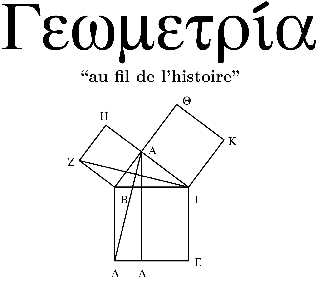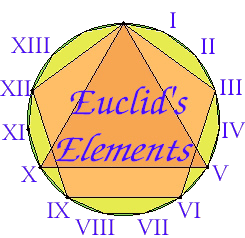Language/LinguaBooks of Mathematics· Book News · Most clicked · Least clicked · Books Index · Search on Amazon

Search for a BookGeometry

 Mathematics -> Geometry Search on Amazon

 Algebraic Geometry Computational Geometry Differential Geometry Discrete geometry Finite Geometry Integral Geometry Noncommutative Geometry Projective Geometry Riemannian Geometry Symplectic Geometry Synthetic Geometry Toric Geometry

EUCLIDS ELEMENTS OF GEOMETRY
Author: J.L. Heiberg  Language:Euclids Elements is by far the most famous mathematical work of classical antiquity, and also has the distinction of being the worlds oldest continuously used mathematical textbook. Little is known . . . . .
Geometric Asymptotics
Author: Victor Guillemin and Shlomo Sternberg  Language:Contents: 1. Introduction: The Method of Stationary Phase 2. Appendix I. Morse's Lemma and Some Generalizations 3. Differential Operators and Asymptotic Solutions 4. Geometrical Optic . . . . .
Comparison Geometry
Author: Edited by Karsten Grove and Peter Petersen  Language:Comparison Geometry asks: What can we say about a Riemannian manifold if we know a (lower or upper) bound for its curvature, and perhaps something about its topology? Powerful results that allow the e . . . . .
Topology and Geometry for Physics
Author: Helmut Eschrig  Language:Contents: Topology; Manifolds; Tensor Fields; Integration, Homology and Cohomology; Lie Groups; Bundles and Connections; Parallelism, Holonomy and Homotopy; Riemannian Geometry; Complex Manifo . . . . .
Modern geometry
Author: Gilbert  Language:Contents: Geometry: Making a Start; Euclidean Parallel Postulate; Non-Euclidean Geometries; Transformations; Inversion.
COURSE OF ANALYTICAL GEOMETRY
Author: RUSLAN A. SHARIPOV  Language:The elementary geometry, which is learned in school, deals with basic concepts such as a point, a straight line, a segment. They are used to compose more complicated concepts: a polygo- nal line, a . . . . .
Foundations of geometry for university students and high-school students
Author: Ruslan Sharipov  Language:This book is a textbook for the course of foundations of geometry. It is addressed to mathematics students in Universities and to High School students for deeper learning the elementary geometry. It c . . . . .
Cours de première année en Géométrie
Author: Gerhard Wanner  Language:Cours de première année en GÉOMÉTRIE, comprenant trois chapitres: I. Géométrie classique, II. Géométrie analytique, III. Géométrie non euclidienne.
A Modern Course on Curves and Surfaces
Author: Richard S. Palais  Language:Contents: Introduction; What is Geometry ; Geometry of Inner-Product Spaces; Linear Maps and the Euclidean Group; Adjoints of Linear Maps and the Spectral ; Differential Calculus on Inner-Product ; . . . . .
Course of linear algebra and multidimensional geometry
Author: Ruslan Sharipov  Language:This is a standard textbook for the course of linear algebra and multidimensional geometry as it was taught in 1991-1998 at Mathematical Department of Bashkir State University. Both coordinate and inv . . . . .
Geometry of Surfaces
Author: Nigel Hitchin  Language:Contents: Topology; Riemann Surfaces; Surfaces in R^3; Hyperbolic Geometry.
Topics in Geometry: Dirac Geometry
Author:   Language:Topics in Geometry: Dirac Geometry
Classical Geometry and Low-Dimensional Topology
Author: Danny Calegari  Language:A continuation of the study of spherical, Euclidean and especially hyperbolic geometry in two and three dimensions. The emphasis will be on the relationship with topology, and the existence of metrics . . . . .
Geometria ed algebra
Author: Prof. Giuseppe Accscina  Language:Dispense di geometria ed algebra, di circa 100 pagine, del corso d\\\'ingegneria informatica, trattano principalmente della teoria degli insiemi, delle funzioni, operazioni fra insiemi e contengono al . . . . .
Sub-Riemannian geometry and Lie groups. Part I
Author: Marius Buliga  Language:These pages covers expository talks during the seminar "Sub-Riemannian geometry and Lie groups" organised by the author and Tudor Ratiu at the Mathematics Department, EPFL, 2001. However, this is the . . . . .
Classical Geometry
Author: Danny Calegari  Language:An introduction to spherical, Euclidean and hyperbolic geometry in two and three dimensions, with an emphasis on the similarities and differences between these flavors of geometry. The most important . . . . .
Euclid\'s Elements
Author:   Language:Euclid\'s Elements form one of the most beautiful and influential works of science in the history of humankind. Its beauty lies in its logical development of geometry and other branches of mathematics . . . . .

```Home |  Authors | About | Contact Us |  Email